# Solution for submission 132236

A detailed solution for submission 132236 submitted for challenge IIT-M RL-ASSIGNMENT-2-TAXI

# What is the notebook about?¶

## Problem - Taxi Environment Algorithms¶

This problem deals with a taxi environment and stochastic actions. The tasks you have to do are:

• Implement Policy Iteration
• Implement Modified Policy Iteration
• Implement Value Iteration
• Implement Gauss Seidel Value Iteration
• Visualize the results
• Explain the results

## How to use this notebook? 📝¶

• This is a shared template and any edits you make here will not be saved.You should make a copy in your own drive. Click the "File" menu (top-left), then "Save a Copy in Drive". You will be working in your copy however you like.

• Update the config parameters. You can define the common variables here

Variable Description
AICROWD_DATASET_PATH Path to the file containing test data. This should be an absolute path.
AICROWD_RESULTS_DIR Path to write the output to.
AICROWD_ASSETS_DIR In case your notebook needs additional files (like model weights, etc.,), you can add them to a directory and specify the path to the directory here (please specify relative path). The contents of this directory will be sent to AIcrowd for evaluation.
AICROWD_API_KEY In order to submit your code to AIcrowd, you need to provide your account's API key. This key is available at https://www.aicrowd.com/participants/me

# Setup AIcrowd Utilities 🛠¶

We use this to bundle the files for submission and create a submission on AIcrowd. Do not edit this block.

In :
!pip install aicrowd-cli > /dev/null


# AIcrowd Runtime Configuration 🧷¶

Get login API key from https://www.aicrowd.com/participants/me

In :
import os

AICROWD_DATASET_PATH = os.getenv("DATASET_PATH", os.getcwd()+"/13d77bb0-b325-4e95-a03b-833eb6694acd_a2_taxi_inputs.zip")
AICROWD_RESULTS_DIR = os.getenv("OUTPUTS_DIR", "results")

In :


API Key valid
Saved API Key successfully!
13d77bb0-b325-4e95-a03b-833eb6694acd_a2_taxi_inputs.zip: 100% 31.2k/31.2k [00:00<00:00, 321kB/s]

In :
!unzip $AICROWD_DATASET_PATH  Archive: /content/13d77bb0-b325-4e95-a03b-833eb6694acd_a2_taxi_inputs.zip replace inputs/inputs_base.npy? [y]es, [n]o, [A]ll, [N]one, [r]ename: A inflating: inputs/inputs_base.npy inflating: inputs/inputs_1.npy inflating: inputs/inputs_0.npy inflating: inputs/inputs_2.npy inflating: targets/targets_2.npy inflating: targets/targets_0.npy inflating: targets/targets_1.npy inflating: targets/targets_base.npy  In : DATASET_DIR = 'inputs/'  ## Taxi Environment¶ Read the environment to understand the functions, but do not edit anything In : import numpy as np class TaxiEnv_HW2: def __init__(self, states, actions, probabilities, rewards, initial_policy): self.possible_states = states self._possible_actions = {st: ac for st, ac in zip(states, actions)} self._ride_probabilities = {st: pr for st, pr in zip(states, probabilities)} self._ride_rewards = {st: rw for st, rw in zip(states, rewards)} self.initial_policy = initial_policy self._verify() def _check_state(self, state): assert state in self.possible_states, "State %s is not a valid state" % state def _verify(self): """ Verify that data conditions are met: Number of actions matches shape of next state and actions Every probability distribution adds up to 1 """ ns = len(self.possible_states) for state in self.possible_states: ac = self._possible_actions[state] na = len(ac) rp = self._ride_probabilities[state] assert np.all(rp.shape == (na, ns)), "Probabilities shape mismatch" rr = self._ride_rewards[state] assert np.all(rr.shape == (na, ns)), "Rewards shape mismatch" assert np.allclose(rp.sum(axis=1), 1), "Probabilities don't add up to 1" def possible_actions(self, state): """ Return all possible actions from a given state """ self._check_state(state) return self._possible_actions[state] def ride_probabilities(self, state, action): """ Returns all possible ride probabilities from a state for a given action For every action a list with the returned with values in the same order as self.possible_states """ actions = self.possible_actions(state) ac_idx = actions.index(action) return self._ride_probabilities[state][ac_idx] def ride_rewards(self, state, action): actions = self.possible_actions(state) ac_idx = actions.index(action) return self._ride_rewards[state][ac_idx]  ## Example of Environment usage¶ In : def check_taxienv(): # These are the values as used in the pdf, but they may be changed during submission, so do not hardcode anything states = ['A', 'B', 'C'] actions = [['1','2','3'], ['1','2'], ['1','2','3']] probs = [np.array([[1/2, 1/4, 1/4], [1/16, 3/4, 3/16], [1/4, 1/8, 5/8]]), np.array([[1/2, 0, 1/2], [1/16, 7/8, 1/16]]), np.array([[1/4, 1/4, 1/2], [1/8, 3/4, 1/8], [3/4, 1/16, 3/16]]),] rewards = [np.array([[10, 4, 8], [ 8, 2, 4], [ 4, 6, 4]]), np.array([[14, 0, 18], [ 8, 16, 8]]), np.array([[10, 2, 8], [6, 4, 2], [4, 0, 8]]),] initial_policy = {'A': '1', 'B': '1', 'C': '1'} env = TaxiEnv_HW2(states, actions, probs, rewards, initial_policy) print("All possible states", env.possible_states) print("All possible actions from state B", env.possible_actions('B')) print("Ride probabilities from state A with action 2", env.ride_probabilities('A', '2')) print("Ride rewards from state C with action 3", env.ride_rewards('C', '3')) base_kwargs = {"states": states, "actions": actions, "probabilities": probs, "rewards": rewards, "initial_policy": initial_policy} return base_kwargs base_kwargs = check_taxienv() env = TaxiEnv_HW2(**base_kwargs)  All possible states ['A', 'B', 'C'] All possible actions from state B ['1', '2'] Ride probabilities from state A with action 2 [0.0625 0.75 0.1875] Ride rewards from state C with action 3 [4 0 8]  ## Task 1 - Policy Iteration¶ Run policy iteration on the environment and generate the policy and expected reward In : # 1.1 Policy Iteration def policy_iteration(taxienv, gamma): # A list of all the states states = taxienv.possible_states # Initial values values = {s: 0 for s in states} # This is a dictionary of states to policies -> e.g {'A': '1', 'B': '2', 'C': '1'} policy = taxienv.initial_policy.copy() ## Begin code here # Hints - # Do not hardcode anything # Only the final result is required for the results # Put any extra data in "extra_info" dictonary for any plots etc # Use the helper functions taxienv.ride_rewards, taxienv.ride_probabilities, taxienv.possible_actions # For terminating condition use the condition exactly mentioned in the pdf while True : while True : delta = 0 for state in taxienv.possible_states : J = values[state] action = policy[state] probabs = taxienv.ride_probabilities(state, action) rewards = taxienv.ride_rewards(state, action) sum = 0 for k in range(len(probabs)) : sum += probabs[k] * (rewards[k] + gamma * values[taxienv.possible_states[k]]) values[state] = sum delta = max(delta, abs(J - values[state])) if delta < 1e-8 : break done = 1 for state in taxienv.possible_states : B = policy[state] temp_array = [] for action in taxienv.possible_actions(state) : probabs = taxienv.ride_probabilities(state, action) rewards = taxienv.ride_rewards(state, action) sum = 0 for k in range(len(probabs)) : sum += probabs[k] * (rewards[k] + gamma * values[taxienv.possible_states[k]]) temp_array.append(sum) policy[state] = taxienv.possible_actions(state)[np.argmax(temp_array)] if policy[state] != B : done = 0; if done == 1 : break # Put your extra information needed for plots etc in this dictionary extra_info = {} """ # Code for printing results string = "" for key in results.keys() : string += "|$\gamma$= " + str(key) + " | A | B | C |" + "\n" string += "|---|---|---|---|\n" for key1 in results[key]['Expected Reward'].keys() : value = results[key]['Expected Reward'][key1] results[key]['Expected Reward'][key1] = round(value, 3) string += "| Expected Reward |" + str(results[key]['Expected Reward']['A']) + "|" + str(results[key]['Expected Reward']['B']) + "|" + str(results[key]['Expected Reward']['C']) + "|\n" string += "| Policy |" + str(results[key]['Policy']['A']) + "|" + str(results[key]['Policy']['B']) + "|" + str(results[key]['Policy']['C']) + "|\n\n___\n\n" print(string) """ ## Do not edit below this line # Final results return {"Expected Reward": values, "Policy": policy}, extra_info  # Task 2 - Policy Iteration for multiple values of gamma¶ Ideally this code should run as is In : # 1.2 Policy Iteration with different values of gamma def run_policy_iteration(env): gamma_values = np.arange(5, 100, 5)/100 results, extra_info = {}, {} for gamma in gamma_values: results[gamma], extra_info[gamma] = policy_iteration(env, gamma) return results, extra_info results, extra_info = run_policy_iteration(env)  # Task 3 - Modifed Policy Iteration¶ Implement modified policy iteration (where Value iteration is done for fixed m number of steps) In : # 1.3 Modified Policy Iteration def modified_policy_iteration(taxienv, gamma, m): # A list of all the states states = taxienv.possible_states # Initial values values = {s: 0 for s in states} # This is a dictionary of states to policies -> e.g {'A': '1', 'B': '2', 'C': '1'} policy = taxienv.initial_policy.copy() ## Begin code here # Hints - # Do not hardcode anything # Only the final result is required for the results # Put any extra data in "extra_info" dictonary for any plots etc # Use the helper functions taxienv.ride_rewards, taxienv.ride_probabilities, taxienv.possible_actions # For terminating condition use the condition exactly mentioned in the pdf H = {s: 0 for s in states} while True : for k in range(m) : for state in taxienv.possible_states : temp_array = [] for action in taxienv.possible_actions(state) : rewards = taxienv.ride_rewards(state, action) probabs = taxienv.ride_probabilities(state, action) sum = 0 for i in range(len(probabs)) : sum += probabs[i] * (rewards[i] + gamma * values[taxienv.possible_states[i]]) temp_array.append(sum) H[state] = max(temp_array) for state in taxienv.possible_states : values[state] = H[state] done = 1 for state in taxienv.possible_states : B = policy[state] temp_array = [] for action in taxienv.possible_actions(state) : rewards = taxienv.ride_rewards(state, action) probabs = taxienv.ride_probabilities(state, action) sum = 0 for i in range(len(probabs)) : sum += probabs[i] * (rewards[i] + gamma * values[taxienv.possible_states[i]]) temp_array.append(sum) policy[state] = taxienv.possible_actions(state)[np.argmax(temp_array)] if policy[state] != B : done = 0 if done == 1 : break # Put your extra information needed for plots etc in this dictionary extra_info = {} ## Do not edit below this line # Final results return {"Expected Reward": values, "Policy": policy}, extra_info  # Task 4 Modified policy iteration for multiple values of m¶ Ideally this code should run as is In : def run_modified_policy_iteration(env): m_values = np.arange(1, 15) gamma = 0.9 results, extra_info = {}, {} for m in m_values: results[m], extra_info[m] = modified_policy_iteration(env, gamma, m) return results, extra_info results, extra_info = run_modified_policy_iteration(env)  In : for key in results.keys() : print(key) print(results[key]['Expected Reward']) print(results[key]['Policy'])  1 {'A': 25.675390625, 'B': 39.27046874999999, 'C': 26.9415625} {'A': '2', 'B': '2', 'C': '2'} 2 {'A': 35.24819580078125, 'B': 48.88519775390625, 'C': 36.4269736328125} {'A': '2', 'B': '2', 'C': '2'} 3 {'A': 51.65408888238907, 'B': 65.30672273488999, 'C': 52.837506109123225} {'A': '2', 'B': '2', 'C': '2'} 4 {'A': 64.95385397075198, 'B': 78.60665630580976, 'C': 66.13728587682095} {'A': '2', 'B': '2', 'C': '2'} 5 {'A': 75.72677981499639, 'B': 89.37958418951888, 'C': 76.91021176751168} {'A': '2', 'B': '2', 'C': '2'} 6 {'A': 84.45285114576716, 'B': 98.10565554580769, 'C': 85.63628309842942} {'A': '2', 'B': '2', 'C': '2'} 7 {'A': 91.52096894114123, 'B': 105.1737733415038, 'C': 92.70440089380395} {'A': '2', 'B': '2', 'C': '2'} 8 {'A': 97.24614435561436, 'B': 110.898948755981, 'C': 98.42957630827706} {'A': '2', 'B': '2', 'C': '2'} 9 {'A': 101.88353644134037, 'B': 115.53634084170707, 'C': 103.0669683940031} {'A': '2', 'B': '2', 'C': '2'} 10 {'A': 105.63982403077847, 'B': 119.29262843114518, 'C': 106.8232559834412} {'A': '2', 'B': '2', 'C': '2'} 11 {'A': 108.68241697822336, 'B': 122.33522137859006, 'C': 109.86584893088607} {'A': '2', 'B': '2', 'C': '2'} 12 {'A': 111.14691726565371, 'B': 124.7997216660204, 'C': 112.33034921831644} {'A': '2', 'B': '2', 'C': '2'} 13 {'A': 113.1431624984723, 'B': 126.79596689883901, 'C': 114.32659445113501} {'A': '2', 'B': '2', 'C': '2'} 14 {'A': 114.76012113705536, 'B': 128.41292553742204, 'C': 115.9435530897181} {'A': '2', 'B': '2', 'C': '2'}  # Task 5 Value Iteration¶ Implement value iteration and find the policy and expected rewards In : # 1.4 Value Iteration def value_iteration(taxienv, gamma): # A list of all the states states = taxienv.possible_states # Initial values values = {s: 0 for s in states} # This is a dictionary of states to policies -> e.g {'A': '1', 'B': '2', 'C': '1'} policy = taxienv.initial_policy.copy() ## Begin code here # Hints - # Do not hardcode anything # Only the final result is required for the results # Put any extra data in "extra_info" dictonary for any plots etc # Use the helper functions taxienv.ride_rewards, taxienv.ride_probabilities, taxienv.possible_actions # For terminating condition use the condition exactly mentioned in the pdf H = {s: 0 for s in states} values_dict = {} iteration = 0 while True : delta = 0 for state in taxienv.possible_states : temp_array = [] for action in taxienv.possible_actions(state) : rewards = taxienv.ride_rewards(state, action) probabs = taxienv.ride_probabilities(state, action) sum = 0 for i in range(len(probabs)) : sum += probabs[i] * (rewards[i] + gamma * values[taxienv.possible_states[i]]) temp_array.append(sum) H[state] = max(temp_array) delta = max(delta, abs(H[state] - values[state])) for state in taxienv.possible_states : values[state] = H[state] values_dict[iteration] = values.copy() if delta < 1e-8 : break iteration += 1 for state in taxienv.possible_states : temp_array = [] for action in taxienv.possible_actions(state) : rewards = taxienv.ride_rewards(state, action) probabs = taxienv.ride_probabilities(state, action) sum = 0 for i in range(len(probabs)) : sum += probabs[i] * (rewards[i] + gamma * values[taxienv.possible_states[i]]) temp_array.append(sum) policy[state] = taxienv.possible_actions(state)[np.argmax(temp_array)] # Put your extra information needed for plots etc in this dictionary extra_info = {'Values_Dict': values_dict} ## Do not edit below this line # Final results return {"Expected Reward": values, "Policy": policy}, extra_info  # Task 6 Value Iteration with multiple values of gamma¶ Ideally this code should run as is In : def run_value_iteration(env): gamma_values = np.arange(5, 100, 5)/100 results = {} results, extra_info = {}, {} for gamma in gamma_values: results[gamma], extra_info[gamma] = value_iteration(env, gamma) return results, extra_info results, extra_info = run_value_iteration(env)  # Task 7 Gauss Seidel Value Iteration¶ Implement Gauss Seidel Value Iteration In : # 1.4 Gauss Seidel Value Iteration def gauss_seidel_value_iteration(taxienv, gamma): # A list of all the states # For Gauss Seidel Value Iteration - iterate through the values in the same order states = taxienv.possible_states # Initial values values = {s: 0 for s in states} # This is a dictionary of states to policies -> e.g {'A': '1', 'B': '2', 'C': '1'} policy = taxienv.initial_policy.copy() # Hints - # Do not hardcode anything # For Gauss Seidel Value Iteration - iterate through the values in the same order as taxienv.possible_states # Only the final result is required for the results # Put any extra data in "extra_info" dictonary for any plots etc # Use the helper functions taxienv.ride_rewards, taxienv.ride_probabilities, taxienv.possible_actions # For terminating condition use the condition exactly mentioned in the pdf ## Begin code here iteration = 0 values_dict = {} while True : delta = 0 for state in taxienv.possible_states : J = values[state] temp_array = [] for action in taxienv.possible_actions(state) : rewards = taxienv.ride_rewards(state, action) probabs = taxienv.ride_probabilities(state, action) sum = 0 for i in range(len(probabs)) : sum += probabs[i] * (rewards[i] + gamma * values[taxienv.possible_states[i]]) temp_array.append(sum) values[state] = max(temp_array) delta = max(delta, abs(J - values[state])) values_dict[iteration] = values.copy() if delta < 1e-8 : break iteration += 1 for state in taxienv.possible_states : temp_array = [] for action in taxienv.possible_actions(state) : rewards = taxienv.ride_rewards(state, action) probabs = taxienv.ride_probabilities(state, action) sum = 0 for i in range(len(probabs)) : sum += probabs[i] * (rewards[i] + gamma * values[taxienv.possible_states[i]]) temp_array.append(sum) policy[state] = taxienv.possible_actions(state)[np.argmax(temp_array)] # Put your extra information needed for plots etc in this dictionary extra_info = {'Values_Dict' : values_dict} ## Do not edit below this line # Final results return {"Expected Reward": values, "Policy": policy}, extra_info  # Task 8 Gauss Seidel Value Iteration with multiple values of gamma¶ Ideally this code should run as is In : def run_gauss_seidel_value_iteration(env): gamma_values = np.arange(5, 100, 5)/100 results = {} results, extra_info = {}, {} for gamma in gamma_values: results[gamma], extra_info[gamma] = gauss_seidel_value_iteration(env, gamma) return results, extra_info results, extra_info = run_gauss_seidel_value_iteration(env)  # Generate Results ✅¶ In : # Do not edit this cell def get_results(kwargs): taxienv = TaxiEnv_HW2(**kwargs) policy_iteration_results = run_policy_iteration(taxienv) modified_policy_iteration_results = run_modified_policy_iteration(taxienv) value_iteration_results = run_value_iteration(taxienv) gs_vi_results = run_gauss_seidel_value_iteration(taxienv) final_results = {} final_results["policy_iteration"] = policy_iteration_results final_results["modifed_policy_iteration"] = modified_policy_iteration_results final_results["value_iteration"] = value_iteration_results final_results["gauss_seidel_iteration"] = gs_vi_results return final_results  In : # Do not edit this cell, generate results with it as is if not os.path.exists(AICROWD_RESULTS_DIR): os.mkdir(AICROWD_RESULTS_DIR) for params_file in os.listdir(DATASET_DIR): kwargs = np.load(os.path.join(DATASET_DIR, params_file), allow_pickle=True).item() results = get_results(kwargs) idx = params_file.split('_')[-1][:-4] np.save(os.path.join(AICROWD_RESULTS_DIR, 'results_' + idx), results)  # Check your local score¶ This score is not your final score, and it doesn't use the marks weightages. This is only for your reference of how arrays are matched and with what tolerance. In : # Check your score on the given test cases (There are more private test cases not provided) target_folder = 'targets' result_folder = AICROWD_RESULTS_DIR def check_algo_match(results, targets): param_matches = [] for k in results: param_results = results[k] param_targets = targets[k] policy_match = param_results['Policy'] == param_targets['Policy'] rv = [v for k, v in param_results['Expected Reward'].items()] tv = [v for k, v in param_targets['Expected Reward'].items()] rewards_match = np.allclose(rv, tv, rtol=3) equal = rewards_match and policy_match param_matches.append(equal) return np.mean(param_matches) def check_score(target_folder, result_folder): match = [] for out_file in os.listdir(result_folder): res_file = os.path.join(result_folder, out_file) results = np.load(res_file, allow_pickle=True).item() idx = out_file.split('_')[-1][:-4] # Extract the file number target_file = os.path.join(target_folder, f"targets_{idx}.npy") targets = np.load(target_file, allow_pickle=True).item() algo_match = [] for k in targets: algo_results = results[k] algo_targets = targets[k] algo_match.append(check_algo_match(algo_results, algo_targets)) match.append(np.mean(algo_match)) return np.mean(match) if os.path.exists(target_folder): print("Shared data Score (normalized to 1):", check_score(target_folder, result_folder))  Shared data Score (normalized to 1): 1.0  ## Visualize results of Policy Iteration with multiple values of gamma¶ Add code to visualize the results In : ## Visualize policy iteration with multiple values of import matplotlib.pyplot as plt # 1.2 Policy Iteration with different values of gamma def run_policy_iteration(env): gamma_values = np.arange(5, 100, 5)/100 results, extra_info = {}, {} for gamma in gamma_values: results[gamma], extra_info[gamma] = policy_iteration(env, gamma) return results, extra_info results, extra_info = run_policy_iteration(env) rewards = {} policies = {} for key in results.keys() : rewards[key] = results[key]['Expected Reward'] policies[key] = results[key]['Policy'] rewards_A = [] rewards_B = [] rewards_C = [] gamma_list = list(results.keys()) for gamma in gamma_list : rewards_A.append(rewards[gamma]['A']) rewards_B.append(rewards[gamma]['B']) rewards_C.append(rewards[gamma]['C']) plt.title('Rewards vs$\gamma$', fontsize = 20) plt.plot(gamma_list, rewards_A, marker = 'o', linestyle = "--", color = 'red', label = "State A") plt.plot(gamma_list, rewards_B, marker = '^', linestyle = "--", color = 'blue', label = "State B") plt.plot(gamma_list, rewards_C, marker = '>', linestyle = "--", color = 'green', label = "State C") plt.legend() plt.xlabel('$\gamma$', fontsize = 15) plt.ylabel('Rewards', fontsize = 15) fig = plt.gcf() fig.set_size_inches(10, 8) plt.show()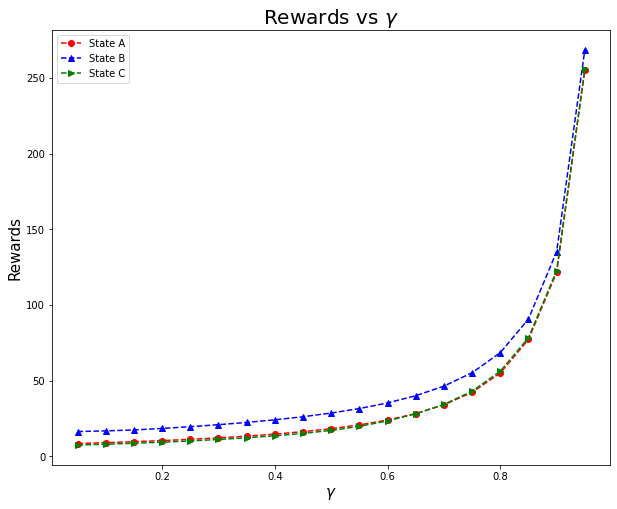# Tabulated policy and reward for each value of$\gamma$¶$\gamma$= 0.05 A B C Expected Reward 8.512 16.4 7.499 Policy 1 1 1$\gamma$= 0.1 A B C Expected Reward 9.077 16.856 8.051 Policy 1 1 1$\gamma$= 0.15 A B C Expected Reward 9.708 17.465 8.669 Policy 1 2 1$\gamma$= 0.2 A B C Expected Reward 10.437 18.482 9.384 Policy 1 2 1$\gamma$= 0.25 A B C Expected Reward 11.274 19.63 10.207 Policy 1 2 1$\gamma$= 0.3 A B C Expected Reward 12.244 20.934 11.163 Policy 1 2 1$\gamma$= 0.35 A B C Expected Reward 13.379 22.431 12.283 Policy 1 2 1$\gamma$= 0.4 A B C Expected Reward 14.722 24.167 13.611 Policy 1 2 1$\gamma$= 0.45 A B C Expected Reward 16.334 26.206 15.207 Policy 1 2 1$\gamma$= 0.5 A B C Expected Reward 18.299 28.636 17.156 Policy 1 2 1$\gamma$= 0.55 A B C Expected Reward 20.79 31.607 19.831 Policy 1 2 2$\gamma$= 0.6 A B C Expected Reward 24.026 35.328 23.459 Policy 1 2 2$\gamma$= 0.65 A B C Expected Reward 28.277 40.096 28.13 Policy 1 2 2$\gamma$= 0.7 A B C Expected Reward 34.062 46.435 34.366 Policy 1 2 2$\gamma$= 0.75 A B C Expected Reward 42.317 55.285 43.106 Policy 1 2 2$\gamma$= 0.8 A B C Expected Reward 55.079 68.558 56.27 Policy 2 2 2$\gamma$= 0.85 A B C Expected Reward 77.247 90.812 78.433 Policy 2 2 2$\gamma$= 0.9 A B C Expected Reward 121.653 135.306 122.837 Policy 2 2 2$\gamma$= 0.95 A B C Expected Reward 255.023 268.765 256.203 Policy 2 2 2 # Subjective questions¶ ## 1.a How are values of$\gamma$affecting results of policy iteration¶ • A higher value of$\gamma$implies a lower probability of the taxi breaking down. We can see in the plots that the expected rewards tend to increase with higher values of$\gamma$. • This is justified as a lower probability of breaking down would imply the taxi driver can keep accumulating rewards for a longer time. • From the graph we can also observe that in the limit that$\gamma \rightarrow 1$, the expected rewards would tend to$\infty$. • With regards to the policy, we observe that initially, the optimal policy is to take action 1 at all states i.e. to cruise the streets looking for passengers. But as$\gamma$increases, we see a shift, the optimal policies for each state shifts to 2 i.e. to goto the nearest taxistand and wait in line. • This is justified by observing the cost and probability matrices. As action 1 is yields high rewards in the short term it is better to take that action when probability of breakdown is high or consequently$\gamma$is low. However, action 2 from all three states A, B, C gives a high probability of moving to town B which is profitable in the long term as we have a very high reward in the transition B$\rightarrow^{2}$B which essentially leads to a high probability chain with a high reward. This action is suitable in the long term when we are ensured that the taxi will not breakdown easily. ## 1.b For modified policy itetaration, do you find any improvement if you choose m=10.¶ ## 1.c Compare and contrast the behavior of Value Iteration and Gauss Seidel Value Iteraton¶ For the sake of the plots below, consider$\gamma = 0.5$. Below we have plots for Values vs Iteration for each of the 3 states A, B, C for both Gauss-Seidel VI and regular VI. ### Observations¶ • For each of the states A, B, and C, we see that the values as obtained by Gauss-Seidel Value Iteration are markedly higher than those obtained by Value Iteration at each iteration/time-stamp • The plots for both VI and Gauss-Seidel VI plataeu at their expected value. But because of the above observation, we see that for all 3 states Gauss-Seidel VI converges to the expected value quicker (at an earlier iteration) than regulat VI. • On repeating the above for$\gamma = 0.1, 0.4, 0.7$and$0.9$, we can observe similar behaviour. In : def plotter(gamma) : gs_results, gs_extra_info = gauss_seidel_value_iteration(env, gamma) results, extra_info = value_iteration(env, gamma) gs_value_dict = gs_extra_info['Values_Dict'] value_dict = extra_info['Values_Dict'] iteration_value_dict = list(value_dict.keys()) iteration_gs_value_dict = list(gs_value_dict.keys()) value = {s: [] for s in ['A', 'B', 'C']} gs_value = {s: [] for s in ['A', 'B', 'C']} for key in iteration_value_dict : for alpha in ['A', 'B', 'C'] : value[alpha].append(value_dict[key][alpha]) for key in iteration_gs_value_dict : for alpha in ['A', 'B', 'C'] : gs_value[alpha].append(gs_value_dict[key][alpha]) fig, axs = plt.subplots(1, 3) axs.plot(iteration_value_dict, value['A'], color = 'red', linestyle = '--', marker = 'o', label = 'VI') axs.plot(iteration_gs_value_dict, gs_value['A'], color = 'red', linestyle = 'solid', marker = 'o', label = 'Gauss-Seidel VI') axs.set_xlabel("Iteration", fontsize = 15) axs.set_ylabel("Values for State A", fontsize = 15) axs.legend(loc = 'lower right', fontsize = 15) axs.set_title("Values vs Iteration for State A for$\gamma$= " + str(gamma), fontsize = 20) axs.plot(iteration_value_dict, value['B'], color = 'blue', linestyle = '--', marker = '^', label = 'VI') axs.plot(iteration_gs_value_dict, gs_value['B'], color = 'blue', linestyle = 'solid', marker = '^', label = 'Gauss-Seidel VI') axs.set_xlabel("Iteration", fontsize = 15) axs.set_ylabel("Values for State B", fontsize = 15) axs.legend(loc = 'lower right', fontsize = 15) axs.set_title("Values vs Iteration for State B for$\gamma$= " + str(gamma), fontsize = 20) axs.plot(iteration_value_dict, value['C'], color = 'green', linestyle = 'dashed', marker = '>', label = 'VI') axs.plot(iteration_gs_value_dict, gs_value['C'], color = 'green', linestyle = 'solid', marker = '>', label = 'Gauss-Seidel VI') axs.set_xlabel("Iteration", fontsize = 15) axs.set_ylabel("Values for State C", fontsize = 15) axs.legend(loc = 'lower right', fontsize = 15) axs.set_title("Values vs Iteration for State C for$\gamma$= " + str(gamma), fontsize = 20) fig = plt.gcf() fig.set_size_inches(40, 10) plotter(0.1) plotter(0.4) plotter(0.5) plotter(0.7) plotter(0.9)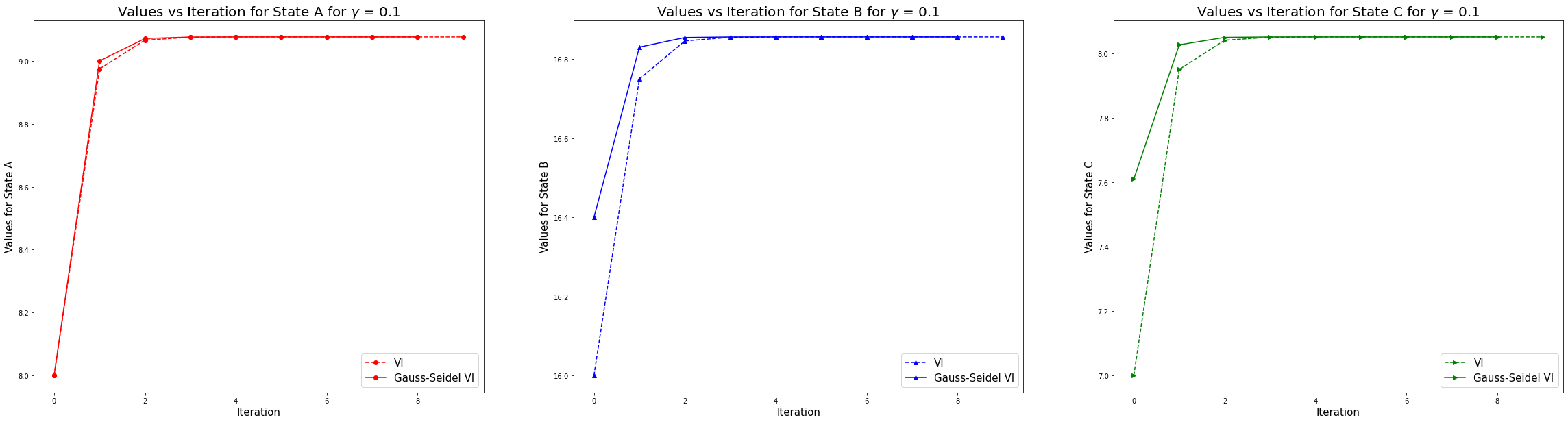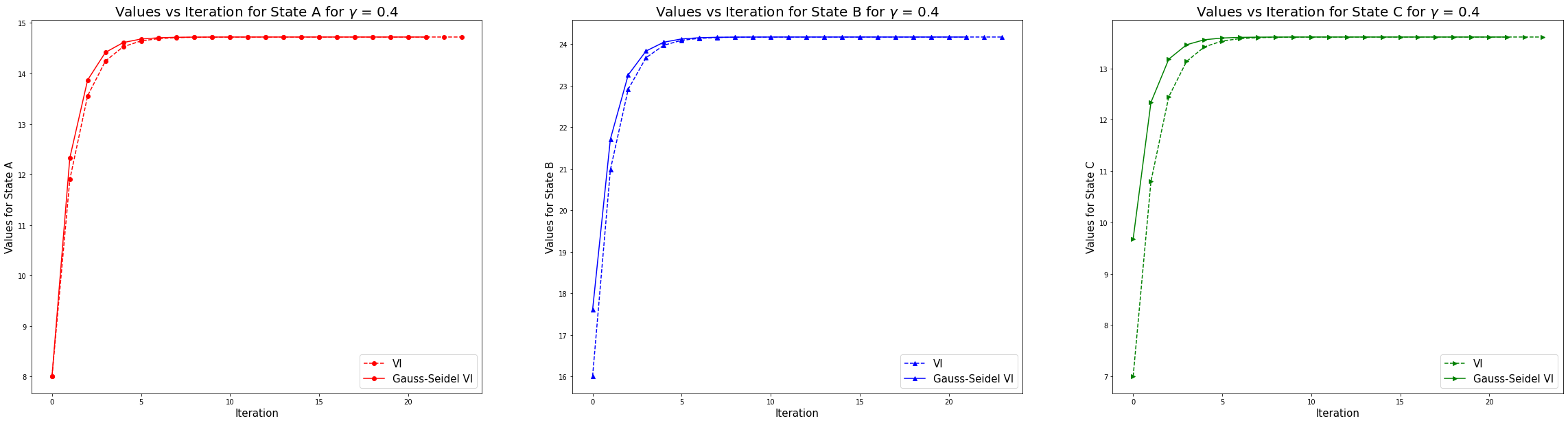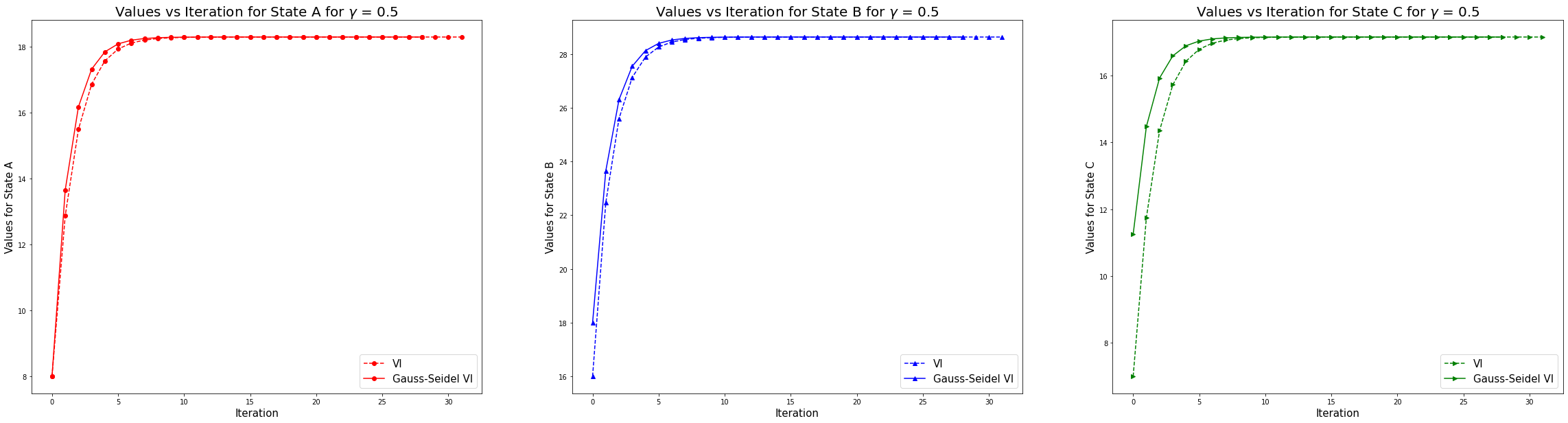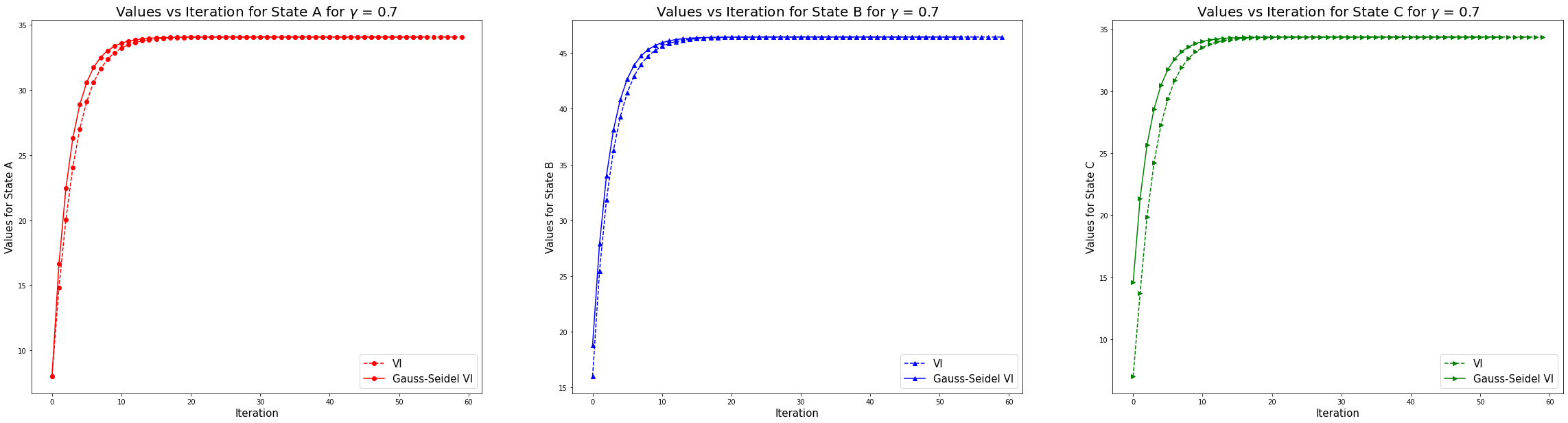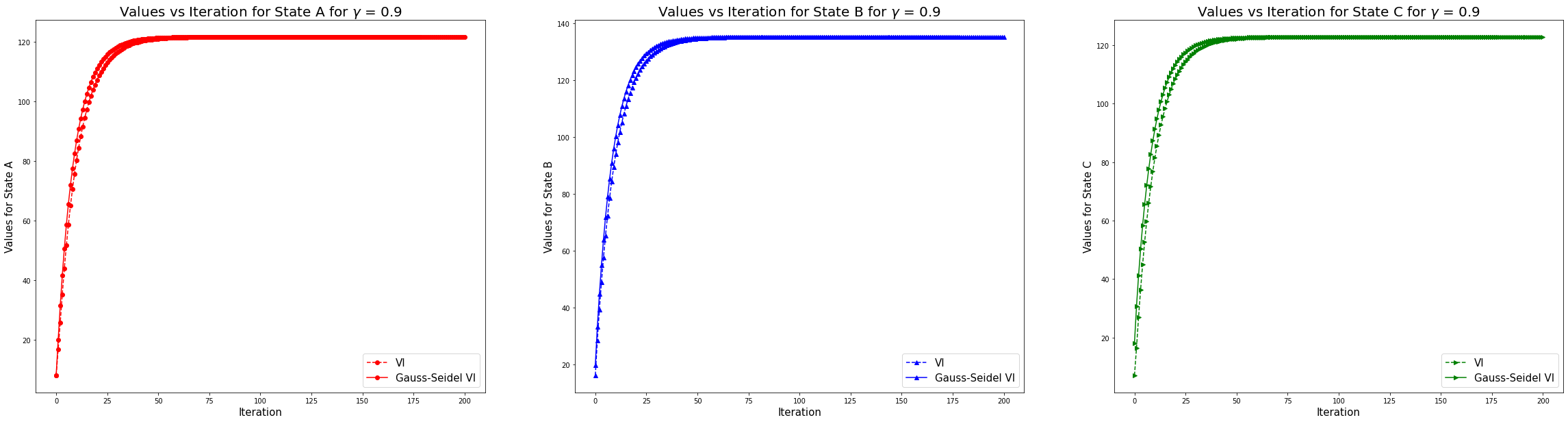# Submit to AIcrowd 🚀¶ In [ ]: !DATASET_PATH=$AICROWD_DATASET_PATH aicrowd notebook submit -c iit-m-rl-assignment-2-taxi -a assets

WARNING: No assets directory at assets... Creating one...
No jupyter lab module found. Using jupyter notebook.

777